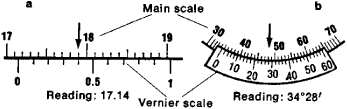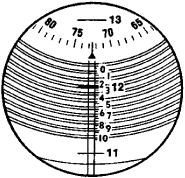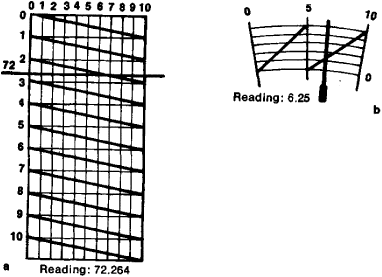# vernier

(redirected from verniers)
Also found in: Dictionary, Thesaurus, Medical.
Related to verniers: vernier caliper, vernier scale

## vernier

vernier (vûrˈnēr), auxiliary scale, either straight or an arc of a circle, designed to slide along a fixed scale. Its unit divisions, usually smaller than those on the fixed scale, permit a far more precise reading. The vernier is attached to the scales of instruments employed for very accurate linear or angular measurements; these include the transit, sextant, barometer, compass, and caliper. It was devised by a French mathematician, Pierre Vernier, who described it in his Construction, usage et propriétés du quadrant nouveau de mathématiques (1631). Certain auxiliary control mechanisms used for fine measurements or adjustments are often called verniers.

## vernier

(ver -nee-er) A short scale used to increase the accuracy of the graduated scale to which it is attached. If nine divisions of the vernier correspond to 10 divisions of the instrument scale, the latter can be read to a further decimal place.
Collins Dictionary of Astronomy © Market House Books Ltd, 2006
The following article is from The Great Soviet Encyclopedia (1979). It might be outdated or ideologically biased.

## Vernier

(1) In instrument making, a device for the exact reading of lengths or angles from the fractional divisions of a scale. The operation of the vernier is based on the eye’s capacity to establish with certainty the coincidence of two lines when one of them is a continuation of the other and their ends overlap. The vernier is a movable scale that can slide along a fixed, basic scale; the divisions on the movable scale are somewhat finer than those on the basic scale. If the interval between the divisions of the basic scale is represented by a, and the interval between divisions on the Vernier is (a - a/n), then the vernier allows the primary scale to be read with an accuracy equal to 1/n of its division. The divisions of the vernier are figured in corresponding fractions of the division of the basic scale. If the zero line of the vernier (index) is located between the two lines c and c + 1 of the basic scale, then the reading is equal to c plus that indication of the vernier which is located opposite the line that best coincides with a certain line of the basic scale.

The vernier was invented in 1631 by P. Vernier (1580-1637), the director of the mint in Franche-Comté, and it was named in his honor.

(2) In radio engineering a device for the exact tuning of radio receivers and other radio equipment.

E. A. IUROV

## Vernier

an auxiliary scale used to read fractions of divisions on the main scale of a measuring device. A prototype of the modern vernier was proposed by the French mathematician P. Vernier. [The Russian term, nonius, comes from the name ofFigure 1. (a) Linear vernier, (b) goniometric vernier

the Portuguese P. Nunes, latinized to Nonius, who proposed a similar device as a means for reading fractions of scale divisions; this device is no longer used.] There are several types of vernier: linear (Figure 1, a), goniometric (Figure 1, b), spiral (Figure 2), and transverse (Figure 3).

The use of a linear vernier is based on a difference in division intervals between the main scale and the vernier scale. The length of the vernier (a whole number of its divisions) is laid out precisely on an integral number of divisions of the main scale. If the zero mark of the vernier coincides with any mark L of the main scale, the measurement result A corresponds to the value given by the mark L. If the zero mark of the vernier does not coincide with L, the value of A becomes A = L + ki, whereFigure 2. Spiral vernier. In the field of vision are the lines of the main millimeter scale (11, 12, 13), one of which is within the zone of ten divisions of the small scale, and also a part of the circular scale, which consists of 100 divisions and two equidistant Archimedean spirals. For reading, a division of the millimeter scale must be in the exact center between two adjacent spirals. Readings are made sequentially on all three scales.

k is the number of vernier divisions from the zero division to the division that coincides with the mark on the main scale, and i is the smallest fraction of a division of the main scale that can be measured with the vernier (usually i = 0.1, 0.05, or 0.02 mm). The principle of reading from a goniometric vernier (used in aFigure 3. Transverse verniers: (a) Linear vernier (transverse scale). A division of the main scale (72) intersects one of the diagonal lines on the vernier. Readings are made according to the location of the point of intersection of the lines and the diagonals. (b) Goniometric vernier; its principle of reading is similar.

number of opticomechanical instruments) is the same as for a linear vernier. The operation of other types of vernier is explained in Figures 2 and 3.

### REFERENCE

Gorodetskii, I. E. Osnovy tekhnicheskikh izmerenii v mashinostroenii. Moscow, 1950.
The Great Soviet Encyclopedia, 3rd Edition (1970-1979). © 2010 The Gale Group, Inc. All rights reserved.

## vernier

[′vər·nē·ər]
(engineering)
A short, auxiliary scale which slides along the main instrument scale to permit accurate fractional reading of the least main division of the main scale.
McGraw-Hill Dictionary of Scientific & Technical Terms, 6E, Copyright © 2003 by The McGraw-Hill Companies, Inc.

## vernier

An auxiliary scale sliding against and used in reading a primary scale; the total length of a given number of divisions on a vernier is equal to the total length of one more or one less than the same number of divisions on the primary scale; makes it possible to read a principal scale much closer than one division of that scale.
McGraw-Hill Dictionary of Architecture and Construction. Copyright © 2003 by McGraw-Hill Companies, Inc.

## vernier

1. a small movable scale running parallel to the main graduated scale in certain measuring instruments, such as theodolites, used to obtain a fractional reading of one of the divisions on the main scale
2. an auxiliary device for making a fine adjustment to an instrument, usually by means of a fine screw thread
Collins Discovery Encyclopedia, 1st edition © HarperCollins Publishers 2005
References in periodicals archive ?
filifera Biozone likely corresponds to the Margachitina elegans global Biozone, as the appearance levels of these species coincide in the range chart of index and characteristic species (Verniers et al.
2003, 2010), as well as the correlation with global chitinozoan biozones (see Verniers et al.
Verniers, J., Nestor, V., Paris, F., Dufka, P., Sutherland, S.
When one is reading an instrument with a vernier, the starting point is the zero line of the vernier.
On a high-quality instrument, the lines are narrow and accurately drawn so it is easy to determine the one line of the vernier that coincides with a line on the main scale.
A variety of vernier scales are used on direct-reading mechanical instruments.
A common error when reading this scale is reading the incorrect side of the vernier. The correct side is determined by the direction of rotation of the instrument (see Figure 7-36).
The vernier scale is a segment and it moves with the telescope of the instrument.
In Figure 7-37, the instrument was rotated clockwise (cw), in the direction of a clock's hands, so the left half of the double vernier scale and the inside angle scale is used.The correct reading is 310[degrees] 32' 0".
In Figure 7-38 the angle scale is being rotated counterclockwise (ccw), the opposite direction of a clock's hands, therefore the outside angle scale and the right half of the vernier scale is used.
Two rules are true when reading double vernier angle scales:

Site: Follow: Share:
Open / Close